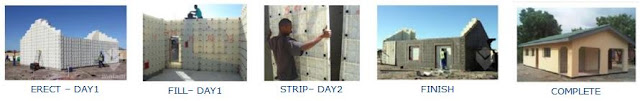COST PER SQUARE METRE

Building cost per square meterCOST PER SQUARE METRE - BRICK & MORTAR OR CONVENTIONAL CONSTRUCTION

Cost per square meter | Building Cost | Construction cost

The shape of a building has an important effect on cost. As a general rule the simpler the shape of a building, the lower will be its unit cost. Let us illustrate this as follows:  This determines the cost per square meter of building

BUILDING SHAPES
Regular shapes in contrast, become more expensive the longer and narrower they are planned. This can be illustrated by the following examples of a square and rectangular building with the same floor area.Cost Per Square Meter

BUILDING A           BUILDING B
Example of how building cost per square meter can vary

BUILDING A
Area on plan = 400m²
Length of walling = 80m
Assume 3m high walling @ Currency 200.00/m²
Then cost/m² = 80 x 3 x 200 ÷ 400
= Currency 120.00/m² - cost per square meter

BUILDING B
Area on plan = 400m²
Length of walling = 208m
Assume 3m high walling @ Currency 200.00/m²
Then cost/m² = 208 x 3 x 200 ÷ 400
= Currency 312.00/m² - cost per square meter

The length of Building B thus resulted in an additional expense of Currency 76,800.00 over that of Building A influencing cost per square meter

Both plans have exactly the same floor areas, yet BUILDING B is far more expensive due to the shape of its design. Reasons for the increase in costs are:

1. Building B has a higher perimeter/floor area ratio (to be discussed in more detail later) and requires 6% more external walling to enclose the same floor area than A.
2. Setting out costs will be increased by as much as 50%.
3. Excavations will cost between 6% and 20% more.
4. Drainage costs will increase by approximately 25% due to the extra manholes and extra length of piping needed.
5. Additional costs will also result from other elements of the building such as the walling and roofing due to the work being complicated by the shape.
It can therefore be concluded that the irregular shapes of buildings add to their overall cost.

SOLUTION TO MANAGE COST

﻿﻿

Read more - Reduce Housing Construction Cost

Keywords - Cost per square metre, moladi, Cost per square meter, shape of a building, Rand, Dollar, R, \$, building, construction, area, house, low cost housing, rate,